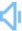Directly to word page Vague search(google)

## Diagram in a sentence

Sentence count:294+13 Only show simple sentencesPosted:2017-01-02Updated:2020-07-24
Synonym: drawSimilar words: Meaning: ['daɪəgræm]n. a drawing intended to explain how something works; a drawing showing the relation between the parts. v. make a schematic or technical drawing of that shows how things work or how they are constructed.Random good picture Not show
1. Draw a simple diagram of the leaf structure.
2. Do you have an installation diagram?
3. Like any such diagram, it is a simplification.
3. Sentencedict.com is a online sentence dictionary, on which you can find good sentences for a large number of words.
4. The lecturer illustrated his point with a diagram on the blackboard.
5. He is drawing a diagram, showing how the machine works.
6. The teacher drew a diagram showing how the blood flows through the heart.
7. This diagram will illustrate what I mean.
8. A diagram represents things in only two dimensions.
9. There's a diagram on page 25.
10. This diagram fits well into the article.
11. The diagram is correct in every detail.
12. The engineer drew a diagram of the bridge.
13. There are explanatory notes with the diagram.
14. The results are shown in diagram 2.
15. On the back of the letter was a diagram.
16. The diagram had been copied with great precision.
17. This is represented in the schematic diagram below.
18. Is this diagram to scale ?
19. A family tree is a diagram with branches, showing how the members of a family are descended and related.
20. The diagram is meant to show the different stages of the process.
21. Compare this system to the one shown in diagram B.
22. You can plot all these numbers on one diagram for comparison.
23. The first diagram is a view of the shop from the street, and the second shows it in section.
24. I'd like you to look at the diagram which is halfway down page 27.
25. a diagram that schematizes the creation and consumption of wealth.
26. Label the diagram clearly.
27. All this information can be conveyed in a simple diagram.
28. The data can be represented graphically in a line diagram.
29. I can't see the relationship between the figures and the diagram.
30. Join the points A and B together on the diagram with a straight line.
Total 294, 30 Per page  1/10  «first  next  last»  goto